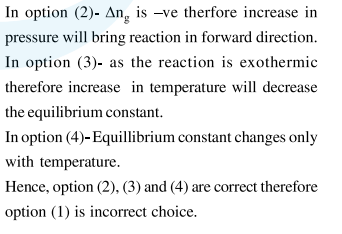# For the reaction,

Question:

For the reaction,

$2 \mathrm{SO}_{2}(\mathrm{~g})+\mathrm{O}_{2}(\mathrm{~g}) \rightleftharpoons 2 \mathrm{SO}_{3}(\mathrm{~g})$

$\Delta \mathrm{H}=-57.2 \mathrm{~kJ} \mathrm{~mol}^{-1}$ and

$\mathrm{K}_{\mathrm{c}}=1.7 \times 10^{16}$

Which of the following statement is INCORRECT?

1. The equilibrium constant is large suggestive of reaction going to completion and so no catalyst is required.

2. The equilibrium will shift in forward direction as the pressure increase.

3. The equilibrium constant decreases as the temperature increases.

4. The addition of inert gas at constant volume will not affect the equilibrium constant.

Correct Option: 1

Solution: